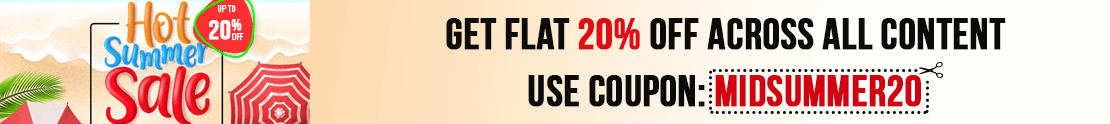# User ForumSubject :IMO    Class : Class 6

Class : Class 1
V=4/3 x Pi x r3
The volume V of a sphere is four-thirds times pi times the radius cubed.
Volume is measured in cubic units.

Class : Class 1
V=4/3 Pi r3
The volume V of a sphere is four-thirds times pi times the radius cubed.
Volume is measured in cubic units.

Subject :IMO    Class : Class 6

## Ans 1:

Class : Class 9

Subject :IMO    Class : Class 6

Class : Class 1

Subject :IMO    Class : Class 4

Class : Class 1
(a) 2

Class : Class 1
(a) 2

## Ans 3:

Class : Class 4
A

Subject :IMO    Class : Class 8

Class : Class 1

Subject :IMO    Class : Class 6

Class : Class 10
c

Class : Class 10

Class : Class 10

## Ans 4:

Class : Class 10
c

Subject :IMO    Class : Class 6

## Ans 1:

Class : Class 8
12 is the answer lo .I am the smartest

## Ans 2:

Class : Class 8
answer shall be equal to 12

## Ans 3:

Class : Class 5
f is a only letter and is 6th so 6/1 is 6. but in lamb there are 4 letters so 12th 1st 2nd and 13th have four letters and so answer must be 28/4 which is 7 and so in the question answer must be 60/5 which i 12. so just check once there might be a mistake .

Class : Class 7

## Ans 5:

Class : Class 7
B IS WROND.. THE CORRECT ANSWER IS 12,12,12,12,12,12,12,12,12,12,12,12,12,12,12,12,12,12,12!!!!!!!!!

Class : Class 7

## Ans 7:

Class : Class 6
60 is not correct answer. F=6 (6th alphabet and only 1 letter therefore (6/1=6)LAMB=7 (12th, 1st, 13th, 2nd alphabet and has 4 letters therefore ((12 1 13 2)/4) ) = (28/4=7).Similarly HOTEL = ((8 15 20 5 12)/5) = (60/5) = 12.correct answer is 12

Class : Class 7

## Ans 9:

Class : Class 9
On terms of the question and solution,we may have two answers, one is 60 and the other is 12.

## Ans 10:

Class : Class 7
The correct answer is 12... as in the word 'LAMB' it is 28 but in the question it said 7 so 28/4 ( as there are 4 letters) is 7.. so in the same way hotel is 60 so 60/5 ( it has 5 letters in it) so the answer is C-12.. there must be a error in the question or in the answer... Please clarify SOF

## Ans 11:

Class : Class 8
THE VALUE FOR LAMB IS DIFFERENT IN THE QUESTION AND SOLUTION

## Ans 12:

Class : Class 10
Yes the answer is 12, if not the value of LAMB must also be 28.

Class : Class 8

## Ans 14:

Class : Class 7
The correct answer is 12 as we have to find the average value of all the letters in the word.

Class : Class 8

## Ans 16:

Class : Class 8

Subject :IMO    Class : Class 6

Class : Class 6

## Ans 2:

Class : Class 6
The answer is (b) First you have add the two numbers 10 and 8 that is 18 Then you have to square the number 18 that is 324

## Ans 3:

Class : Class 7
Correct a bit tricky

Subject :IMO    Class : Class 4

Class : Class 4
A

## Ans 2:

Class : Class 8
a

Subject :IMO    Class : Class 7

## Ans 1:

Class : Class 7
Wrong Question if not wrong explain it properly

## Ans 2:

Class : Class 7
pls explain as the side is not provided

## Ans 3:

Class : Class 9
pl explain the steps to solve this question

## Ans 4:

Class : Class 7
pls explain in detail

Class : Class 7

## Ans 6:

Class : Class 9
side of the square isnt given /wrong question